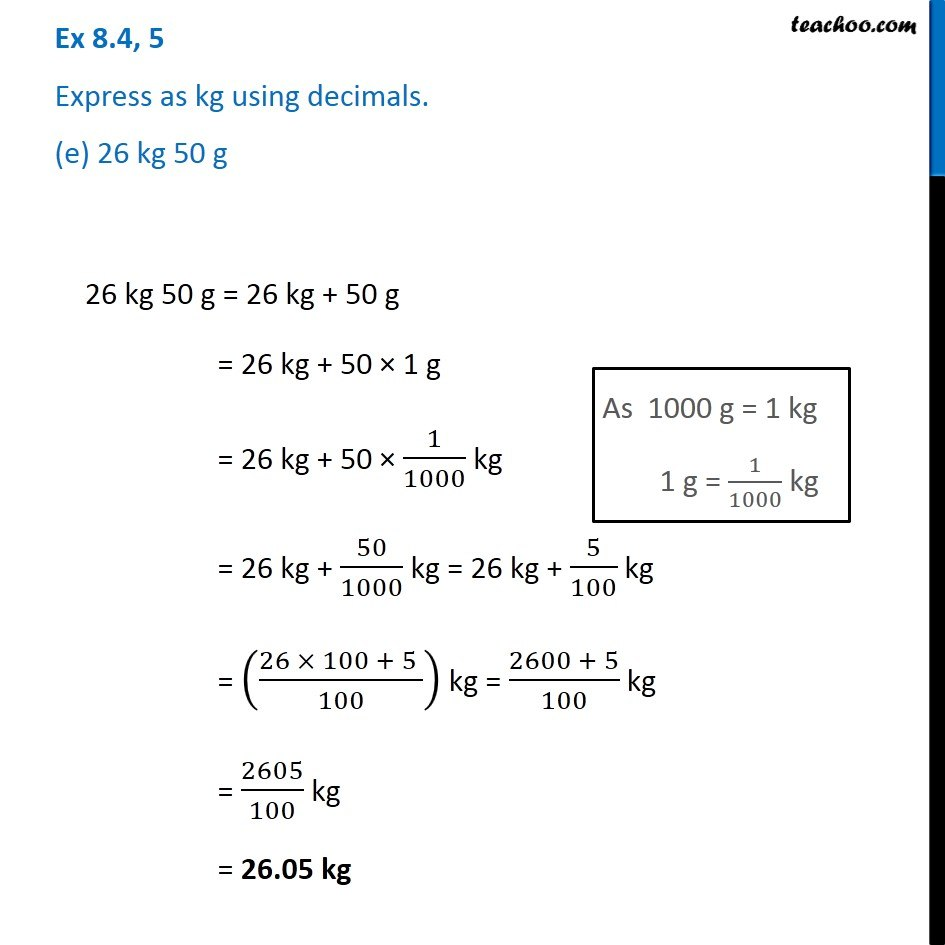1. Chapter 8 Class 6 Decimals
2. Serial order wise
3. Ex 8.4

Transcript

Ex 8.4, 5 Express as kg using decimals. (e) 26 kg 50 g 26 kg 50 g = 26 kg + 50 g = 26 kg + 50 × 1 g = 26 kg + 50 × 1/1000 kg = 26 kg + 50/1000 kg = 26 kg + 5/100 kg = ((26 × 100 + 5 )/100) kg = (2600 + 5)/100 kg = 2605/100 kg = 26.05 kg